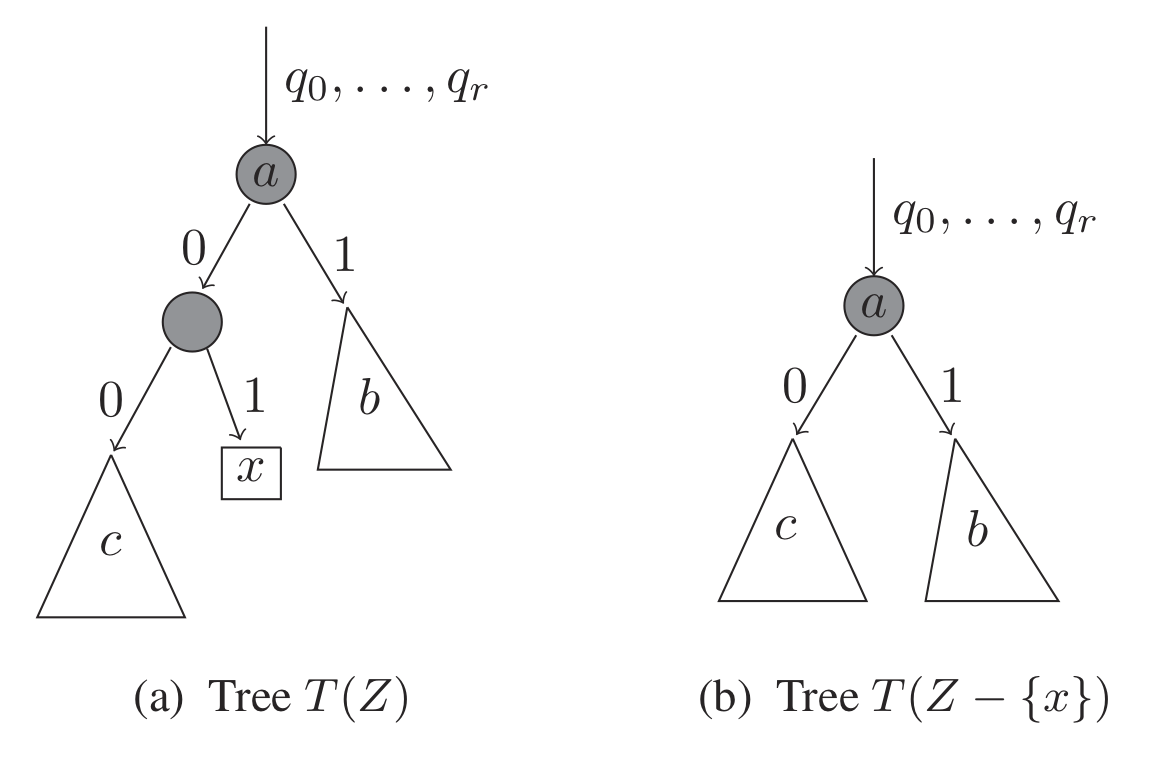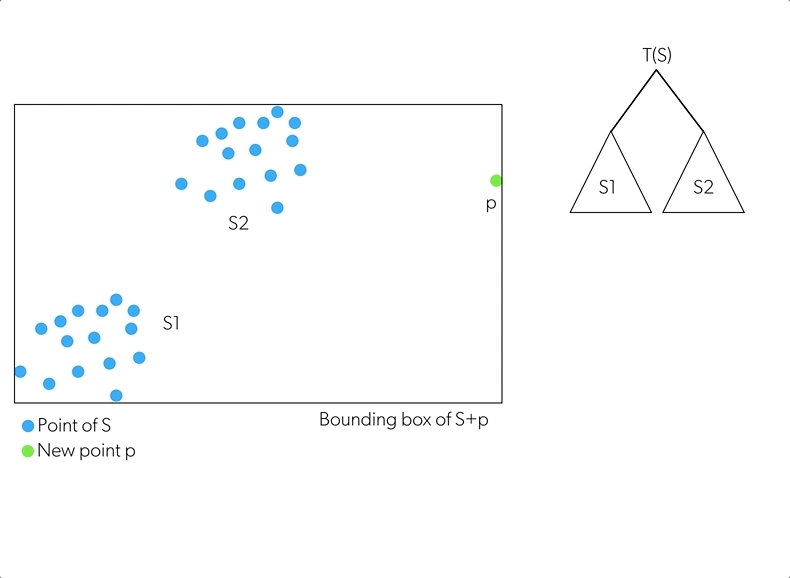Home

# Anomaly detection in hostile environment

## Streaming Machine Learning to detect anomalies

By MFG Labs
04/25/2019
How streaming machine learning can be used to help companies implement systems that detect anomalies among millions of events.Context

For a TV broadcaster client, we had to design a outlier detection system, able to handle hundreds of millions of events per day. Outlier detection is not a new topic, but being able to apply it to « wild » data streams, with a minimum parametrization, is another challenge, at the crossroads between Machine Learning and Streaming Data Infrastructure. The topic is becoming more and more important with the advent of IoT. From an algorithmic standpoint, there’s even a name for that : Streaming Machine Learning. One key point is being able to do computations with a given amount of memory. This article aims to explain an original algorithm for anomaly detection, developed by Amazon Web Services (AWS), and how it fits in the ecosystem of existing anomaly detection algorithms.

# What is anomaly detection?

Outliers in a dataset are entries that don’t fit in with the others, that are well outside of the expected range of values. As such, they need to be handled carefully for direct use (monitoring) or during preprocessing (machine learning pipeline); even a classification task can be turned into an outlier detection problem if the classes are highly skewed. Those entries represent states that shouldn’t be observed and therefore are also called anomalies. Usually due to the size of the data and the way the data is collected, we can’t label the points as anomalous or normal and unsupervised techniques need to be used.

There is a wide range of algorithms used for anomaly detection:

• Based on distribution and quantiles (z-score, t-digest)
• Based on density (DBSCAN)
• Based on isolation trees (iForest, RCF)
• For times series: classic algorithms that don’t take into account the temporality of the data, as well as predictive algorithms. For the latter, we consider a point as normal if its prediction is « close enough » to the real value, and as anomalous otherwise.

# A focus on isolation-based algorithms

Isolation-based anomaly detection algorithms don’t rely on similarity between points but rather on the fact that anomalous points are sparse and different from normal points distribution, they can thus be isolated. In order to reduce the running time and the memory used, these algorithms make some random decisions regarding feature choice, which explains their popularity when dealing with high-dimensional data or when implementing a streaming application.

iForest

The first algorithm to use that assumption was iForest. The aim is to build a forest of binary trees.Each tree is built on a subset of the available data. We randomly select a dimension, do a random cut along this dimension (between the min and the max of this dimension) and repeat on the two resulting subsets until all the points are isolated i.e. are in a leaf.

The closer a point is to the root of a tree, the more it is considered as an anomaly. Therefore the depth of a point from the root is used to compute a score between 0 and 1 (0 normal point, 1 anomalous point).

Robust Random Cut Forest (RRCF)

Researchers from AWS, Stanford University and the University of Pennsylvania took this method one step further to create the Robust Random Cut Forest (RRCF) algorithm.

The construction of a tree is basically the same as for iForest except that the dimension on which the cut is done is not purely random: the probability of a dimension being chosen is proportional to the spread of the data on that dimension. It means that at each step the algorithm optimises (to some extent) the dimension on which the cut is done. It results in a more efficient algorithm. The score is different though but follows the same principle: high score = anomaly, low score = normal point.Example of the construction of a tree

Let’s focus on a central concept in the RCF algorithm : the notion of displacement.

Definition of the displacement of a point and a tree :• Z is the original set of points
• Z — {x} is the original set, without point x
• x the point for which we want to compute the displacement
• T a tree of the forest
• f(y,Z,T(Z)) is the depth of the point y in the tree T built on Z.
• Pr[T] is

Example

Let’s look at the displacement of a new point x. It can be viewed as the increase of complexity of the tree in the presence of x:When we look at the two trees (with and without the point x), we see that all the points in the subtree c have their depth reduced by one in the tree without x while the points in the subtree b have their depth unchanged. The displacement of the point x for this tree will be proportional to the number of points in the subtree c.

This formula could work to discriminate individual outliers from normal points:

When considering a dense cluster of points and a single outlier p far-off, a tree built on this dataset is likely to have a root node with the outlier on one side and the cluster on the other side. The displacement of the outlier is then proportional to the number of points in the cluster which is substantial.

But it doesn’t generalize well to a group of outliers (far from the normal points but close to each other) because of the masking effect:

When looking at the previous diagram, if we consider that a is the root, x an outlier and c a group of outliers close to x, the displacement of the point x will be proportional to the number of points in c (as stated previously) and since there are few outliers in general this score will be low.

To palliate this, the authors extended the formula to a subsample of points containing x which they called the collusive displacement.

Definition for a point x and the forest of trees

CODISP(x,Z)=ESZ,T[xCS1∣C∣∑yS-C(f((y,S,T(S))-f(y,S-C,T(S-C)))]

• Z is the whole set of points
• S is a subset on which the tree T is built
• x is the point for which we want to compute the displacement
• C is a subset of S containing x
• f(y,S,T(S)) is the depth of the point y in the tree T build on Sz

It handles efficiently the case of a group of outliers and gives a high score to each outlier. If we take the previous example and instead of computing the displacement of x we compute the displacement of x and c, the score will be proportional to the number of points in the subtree b thus significant (since we consider the points composing b to be normal so in large number).

In order to compute this score, it is mandatory for the point to be in the tree. Therefore we need to insert the point if it is not already the case: we want to insert the point p into the tree T built on points S. We do a random cut on a random dimension considering the bounding box of S+p. If the cut separates p from S, the new tree has a root node with the tree T on one hand and p on the other hand (first GIF below).If it is not the case, we don’t use this cut but we use the first cut of the tree T instead (which separates S into S1 and S2) and we do the procedure again, this time considering the point p and the subset which falls on the same side as p (GIF below).Shingling

While working on one-dimensional time-series, the authors noticed that the algorithm was performing better if a shingle was used: the point at t is represented by a vector of dimension K (for a shingle of size K) instead of a vector of dimension one. The first component is the value a t, the second component is the value at t-1, …, the last component is the value at t-(K-1). Instead of feeding the RCF with one-dimensional data, we use K-dimensional data as input.

This score is said to be more efficient than the one developed in iForest: The RRCF performs better on datasets with quasi-constant dimensions as well as when the anomalous points are not present in the training dataset. When comparing the results on time series, the authors also found that their algorithm better detects the beginning of an anomalous period of time.

RRCF Implementation

The algorithm is available only in AWS, but both in SageMaker (batch processing) and Kinesis Analytics (streaming processing).

In SageMaker, through a Jupyter notebook, we can call the function by simply loading the SageMaker library.

In Kinesis Analytics, the function works on a data stream and return a stream of scores.

For both implementations, the parameters are the number of trees in the forest, the size of the sample to build each tree and the shingle size; there is a fourth parameter in Kinesis Analytics — the time decay — that represents the number of previous points that we take into account (the faster a time series is changing the smaller the time decay is advised to be).

## Pros:

• Optimized for streaming processing
• Non parametric algorithm (no assumption on the distribution of the data)
• No preprocessing (maybe useful for edge computing ?)
• Speed
• Few parameters to optimize

NB: The last 3 are common with all isolation-based algorithms

## Cons:

• Only available on AWS
• Different implementations (if on SageMaker or Kinesis Analytics)
• Poor interpretability of result (black box)

# Conclusion

Isolation Forests are efficient algorithms which are designed to directly identify outliers. The data structure used for this is a forest of binary trees. The added values of RRCF compared to iForest are the effective construction of the trees with stream data, the handling of irrelevant dimensions and the scoring in a stream.

The « zero parameterization » approach makes it quite robust and easy to setup and maintain. Its output (normalized anomaly score) could also be taken as input for more complex approaches, combining long term memory and multivariate data. In that vein, we tried to couple RCF with another AWS algorithm: Deep AR, but that’s another story!

See other news